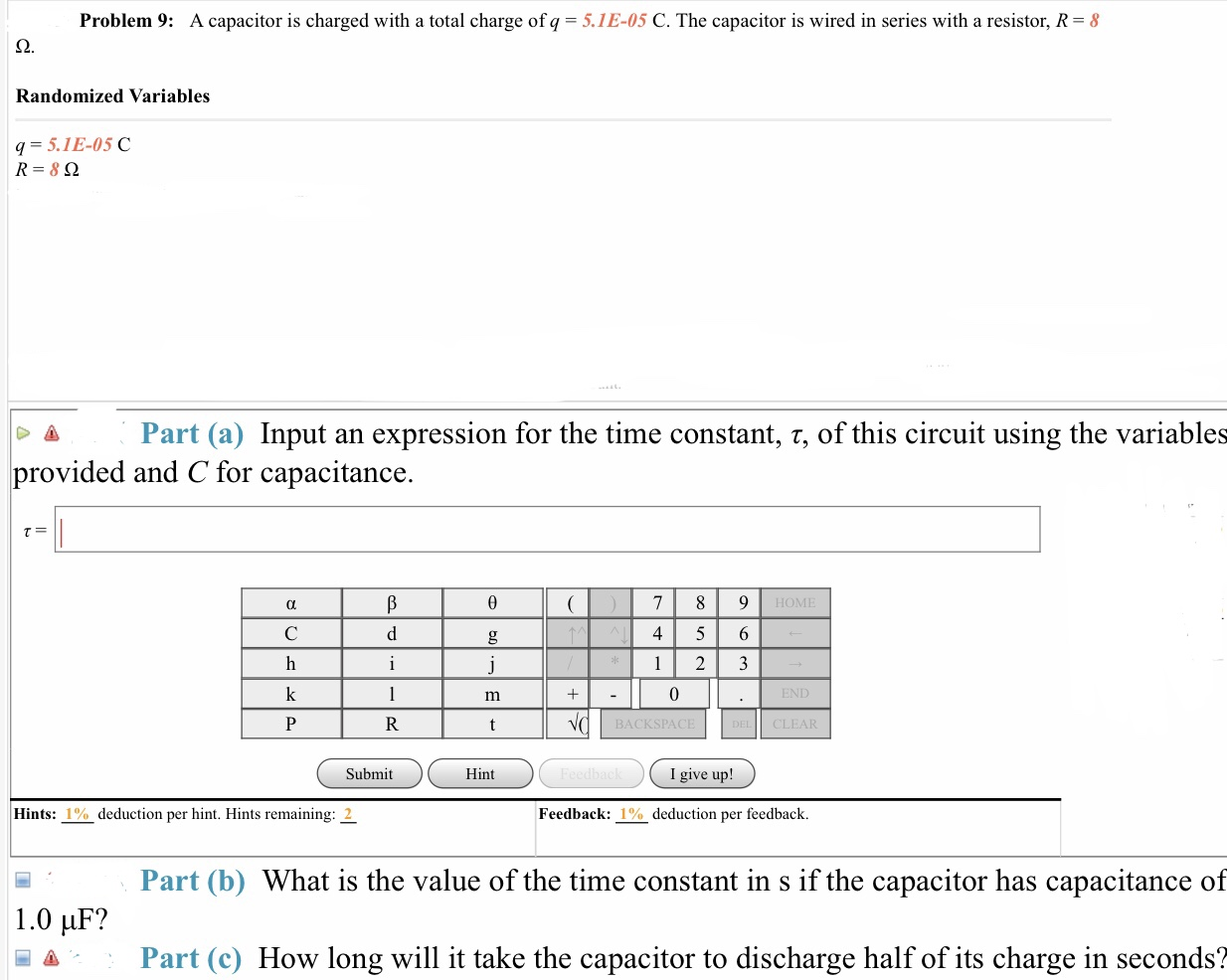# Problem 9: A capacitor is charged with a total charge of q = 5.1E-05 C. The capacitor is wired in series with a resistor, R-8 Randomized Variables q = 5.1 E-05 C ← Δ Part (a) Input an expression for the time constant, τ, of this circuit using the variables provided and C for capacitance. HOME 4 5 6 BACKS CLEAR Submit Hint I give up! Hints: 1 -deduction per hint. Hints remaining: Feedback: deduction per feedback. Part (b) What is the value of the time constant in s if the capacitor has capacitance of 1.0 μF? Δ Part (c) How long will it take the capacitor to discharge half of its charge in seconds?

Questionhelp_outlineImage TranscriptioncloseProblem 9: A capacitor is charged with a total charge of q = 5.1E-05 C. The capacitor is wired in series with a resistor, R-8 Randomized Variables q = 5.1 E-05 C ← Δ Part (a) Input an expression for the time constant, τ, of this circuit using the variables provided and C for capacitance. HOME 4 5 6 BACKS CLEAR Submit Hint I give up! Hints: 1 -deduction per hint. Hints remaining: Feedback: deduction per feedback. Part (b) What is the value of the time constant in s if the capacitor has capacitance of 1.0 μF? Δ Part (c) How long will it take the capacitor to discharge half of its charge in seconds? fullscreen

### Want to see this answer and more?

Experts are waiting 24/7 to provide step-by-step solutions in as fast as 30 minutes!*

*Response times vary by subject and question complexity. Median response time is 34 minutes and may be longer for new subjects.
Tagged in
SciencePhysics

### Alternating Current